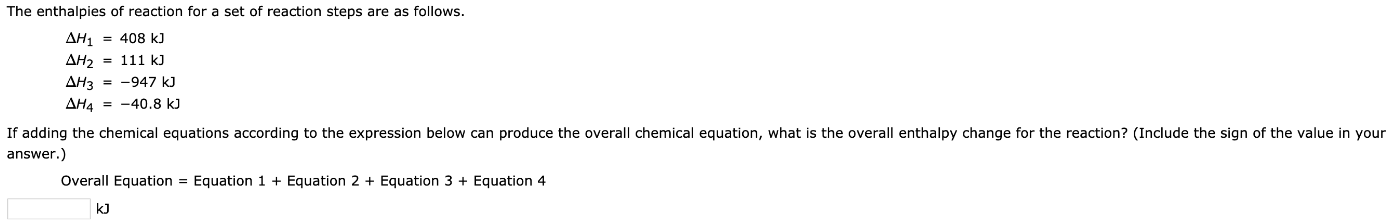# The enthalpies of reaction for a set of reaction steps are as follows. ΔH1 = 408 kJ ΔH2 = 111 kJ ΔH3 = -947 kJ ΔH4 = -40.8 kJ If adding the chemical equations according to the expression below can produce the overall chemical equation, what is the overall enthalpy change for the reaction? (Include the sign of the value in your answer.) Overall equation = Equation 1 + Equation 2 + Equation 3 + Equation 4